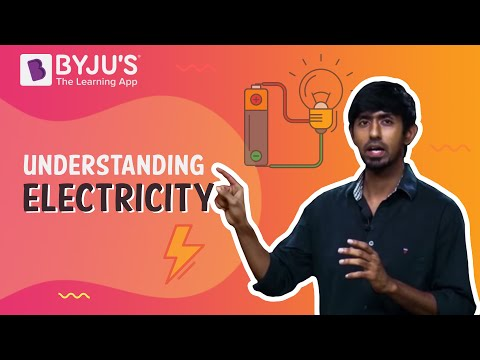# Electric Current Questions

Electric current is the movement of charges through a conducting medium with respect to time. In Physics, electric current is defined as the flow of charged particles, such as electrons moving through an electrical conductor. The higher the voltage or potential difference, the more the current flow.

The SI unit for current is ampere or amp, denoted by A.
1 Ampere = 1 Coulomb/1Second

Types of Electric Current – Electric current is of two types, alternating current (AC) and direct current (DC). The direct current flow is unidirectional, whereas the flow of alternating current is bidirectional. Direct current is mostly used in low voltage applications such as battery charging. Alternating current is used to run appliances either industrial or commercial.

## Important Questions on Electric Current with Answers

1) Which among the following factors does the electrical resistivity of a metallic wire depend on?

(a) Length

(b) Thickness

(c) Nature of the material

(d) Its shape

Explanation: Resistivity is an intrinsic property, therefore it does not depend on the geometry of the material, rather it depends on the nature of the material.

2) Which among the following materials allow larger currents to flow through them?

(a) Insulators

(b) Conductors

(c) Alloys

(d) Semiconductors

Explanation: Conductors have free electrons which start moving when it’s connected to a battery and therefore, conductors can also be termed as free charge carriers.

3) The hindrance offered by the material of conductor to the smooth flow electric current is known as:

(a) Resistance

(b) Inductance

(c) Conductance

(d) None of these

Explanation: Resistance is a property of a conductor, due to which it resists the flow of electric current through it.

4) The quantity of work done in joules, in moving the one-unit electric charge from one point to another point in an electric circuit is termed as:

(a) Electric Resistance

(b) Electric Current

(c) Electric Conductance

(d) Potential Difference

Explanation: Potential difference is defined as the amount of work done in moving a unit charge from one point to another, where the work done will be in joules.

5) When an electric current is passed, the movement of electrons takes place from

(a) Higher potential to lower potential

(b) Lower potential to higher potential

(c) Out of the conductor

(d) Do not move

Explanation: The higher terminal has a higher number of positive charges and the lower potential terminal has more negative charges and also the electrons are mobile, as a result, electrons from low-potential terminals travel to higher-potential terminals.

6) The commercial unit of electrical energy is

(a) Watt-hour

(b) Kilowatt-hour

(c) Kilojoules

(d) Joules

Explanation: The amount of energy consumed by a machine working at a rate of 1KW per hour is termed as KW-hour, the commercial unit of electrical energy.

7) Electric power is inversely proportional to

(a) Voltage

(b) Resistance

(c) Temperature

(d) Current

Explanation: Since, Power (P) = V2/R therefore it is inversely proportional to the resistance.

8) For confirmation of Ohm’s Law, which among the following points should be kept in mind?

(a) Ammeter and voltmeter should be linked in series

(b) Ammeter and voltmeter should be linked in parallel

(c) Ammeter should be connected in parallel and voltmeter in series

(d) Voltmeter should be connected in parallel and ammeter in series

Explanation: An ammeter is a low resistance device hence rather than connecting it in parallel, it is connected in series so that it could draw zero current from the circuit. On the other hand, a voltmeter is a device of higher resistance, hence it is connected parallel to the circuit to avoid resistance in the flow of current in the circuit.

9) Which of the following instruments is used to measure electric potential?

(a) Ammeter

(b) Voltmeter

(c) Potentiometer

(d) Galvanometer

Explanation: A voltmeter is a device that measures the potential difference between two points in an electric circuit.

10) What is the purpose of rheostat?

(a) Decreases the magnitude of current only

(b) Increases the magnitude of current only

(c) Increases or decreases the magnitude of current

(d) None of these

Explanation: A rheostat is a type of variable resistor that can be used to change the magnitude of current without interrupting its flow in a circuit.

### Practice Questions

1. An electric bulb is connected to a 220V generator. The current is 0.50 A. What is the power of the bulb?
2. Find the value of the resistance of the resistor, when a 40V battery is linked across an unknown resistor and there is a current of 100 mA in the circuit.
3. Define electric potential.
4. What is meant by the potential difference between the two points?
5. Define one-ampere electric current.

Understand Electricity in just 5 minutes by watching the video.